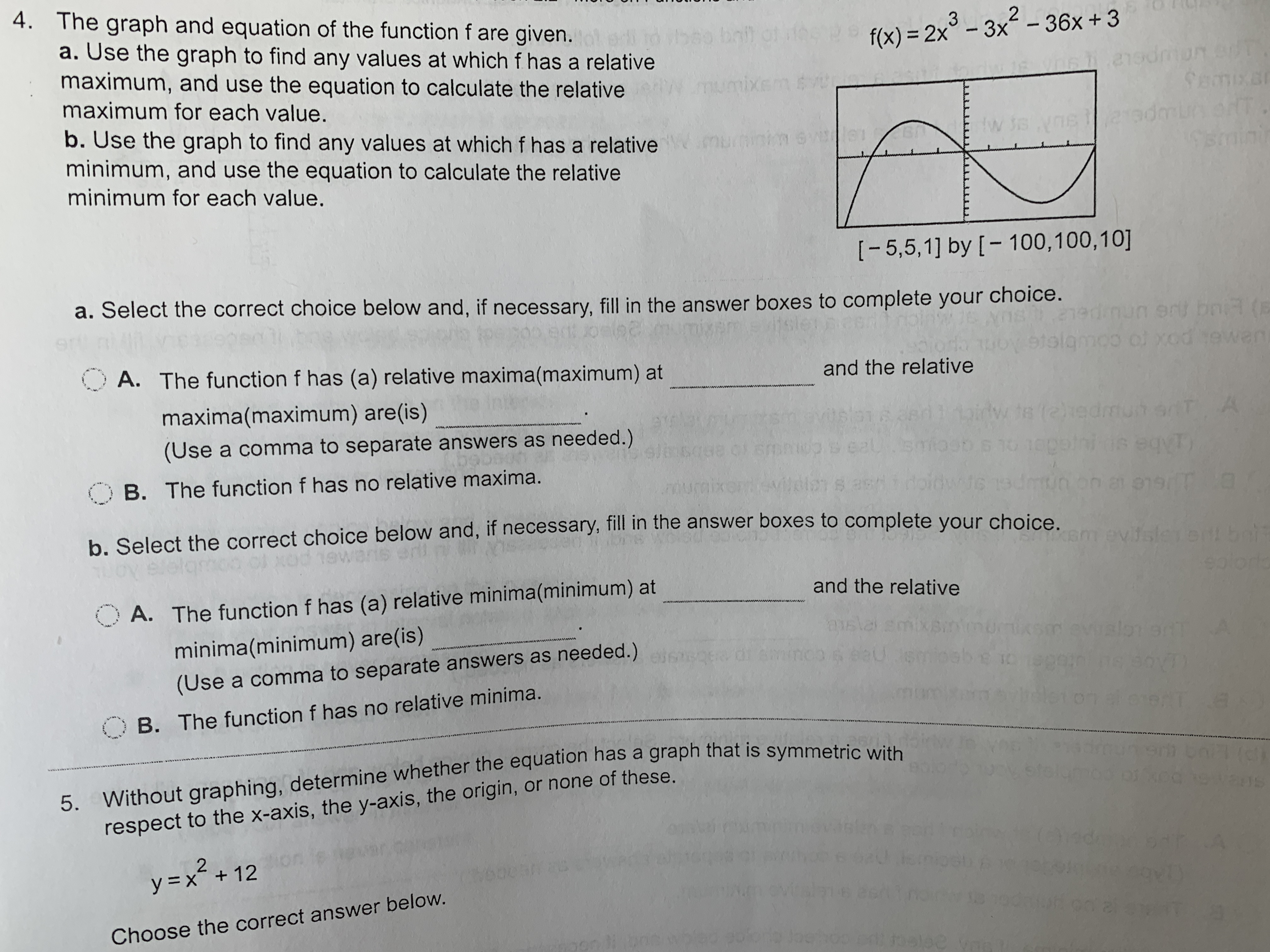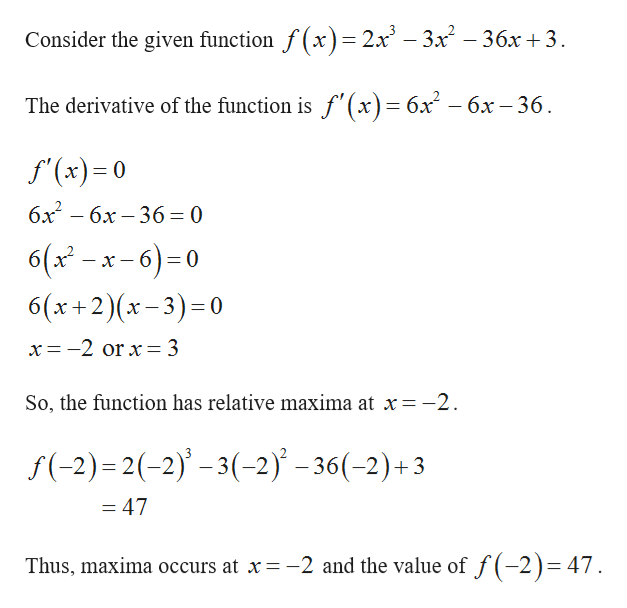# 4. The graph and equation of the function f are given.a. Use the graph to find any values at which f has a relativemaximum, and use the equation to calculate the relative32O1bot ot faf(x) 2x-3x-36x +3nsdo etSemxardmu anT.minimaximum for each value.wixemb. Use the graph to find any values at which f has a relativeminimum, and use the equation to calculate the relativemuiniminimum for each value.[-5,5,1] by [- 100,100,10]a. Select the correct choice below and, if necessary, fill in the answer boxes to complete your choice.edimun ert bn (E1olamoo of yod tewan.OA. The function f has (a) relative maxima(maximum) atand the relativemaxima(maximum) are(is)nyvitsionTAw ts (e)hedmut ariT Aeqya(Use a comma to separate answers as needed.)6pnidOB. The function f has no relative maxima.lonb. Select the correct choice below and, if necessary, fill in the answer boxes to complete your choiceevisieeolortaand the relativeA. The function f has (a) relative minima(minimum) atwww.wwwaslaco &eauks evualoner9 1I0minima(minimum) are(is)(Use a comma to separate answers as needed.)984i e soviTYB. The function f has no relative minima.5. Without graphing, determine whether the equation has a graph that is symmetric withrespect to the x-axis, the y-axis, the origin, or none of these.2y x + 12ARChoose the correct answer below.nt wpeobetoo anil foelee

Question

Can someone help me solve this problem? It is number fourhelp_outlineImage Transcriptionclose4. The graph and equation of the function f are given. a. Use the graph to find any values at which f has a relative maximum, and use the equation to calculate the relative 3 2 O1 bot ot fa f(x) 2x-3x-36x +3 nsdo et Semxar dmu anT. mini maximum for each value. wixem b. Use the graph to find any values at which f has a relative minimum, and use the equation to calculate the relative muini minimum for each value. [-5,5,1] by [- 100,100,10] a. Select the correct choice below and, if necessary, fill in the answer boxes to complete your choice. edimun ert bn (E 1olamoo of yod tewan. OA. The function f has (a) relative maxima(maximum) at and the relative maxima(maximum) are(is) ny vitsion TA w ts (e)hedmut ariT A eqy a (Use a comma to separate answers as needed.) 6pnid OB. The function f has no relative maxima. lon b. Select the correct choice below and, if necessary, fill in the answer boxes to complete your choice evisie eolorta and the relative A. The function f has (a) relative minima(minimum) at www.www asla co &eau ks evualoner 9 1I0 minima(minimum) are(is) (Use a comma to separate answers as needed.) 984i e soviTY B. The function f has no relative minima. 5. Without graphing, determine whether the equation has a graph that is symmetric with respect to the x-axis, the y-axis, the origin, or none of these. 2 y x + 12 AR Choose the correct answer below. nt wpeo betoo anil foelee fullscreen
check_circle

Step 1

The given function is f(x) = 2x3–3x2–36x+3.

Find the maxima and minima of the function by diffe...help_outlineImage TranscriptioncloseConsider the given function f(x)= 2x - 3x - 36x +3 The derivative of the function is f'(x)= 6x - 6x -36 f'(x) 0 бх — бх — 36 — 0 6(xx-6)0 6(x+2)(x-3) 0 x = -2 or x= 3 So, the function has relative maxima at x=-2 f(-2) 2(-2)-3(-2) -36(-2)+3 = 47 Thus, maxima occurs at x-2 and the value of f(-2)= 47. fullscreen

### Want to see the full answer?

See Solution

#### Want to see this answer and more?

Solutions are written by subject experts who are available 24/7. Questions are typically answered within 1 hour.*

See Solution
*Response times may vary by subject and question.
Tagged in

### Other